Physics
Fox

### Summary

• Light reflecting off a flat mirror (aka. a plane mirror) looks like this: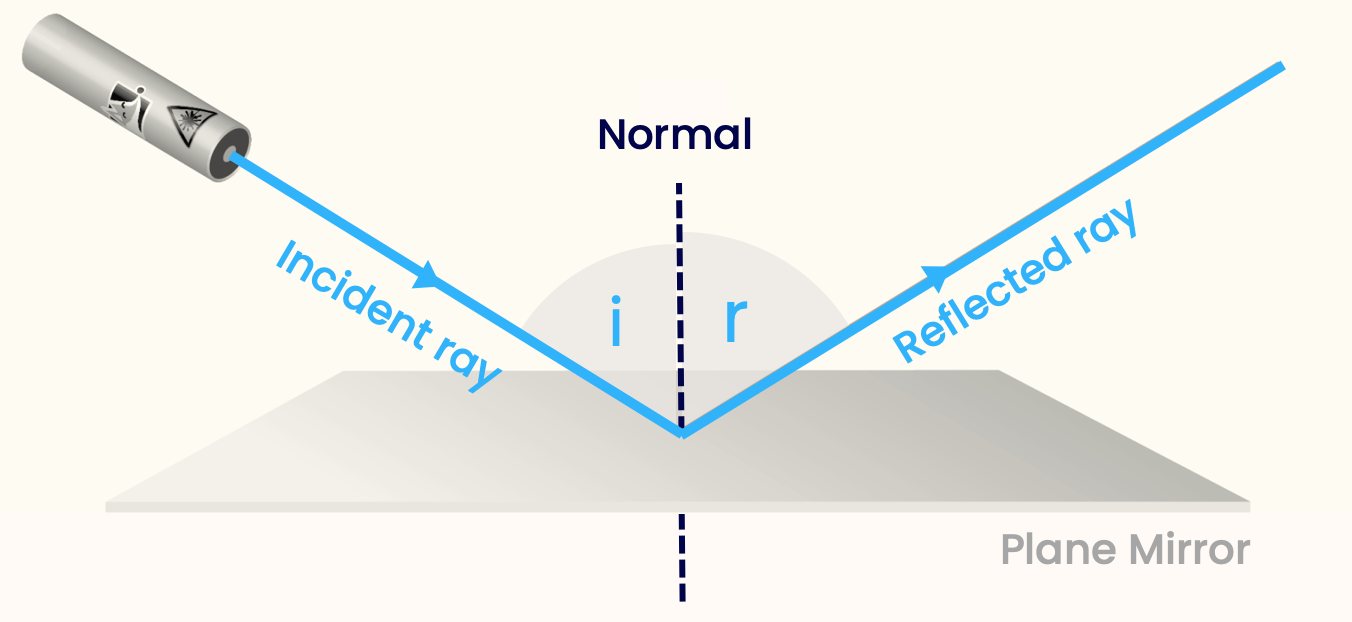NB: Angles $$i$$ and $$r$$ are between the light ray and the normal (the dotted line). The normal is always at right-angles the mirror.

• The Law of Reflection: When light reflects off a plane mirror, the angle of incidence $$i$$ is always the same as the angle of reflection $$r$$
We can write this as an equation: $i = r$

Thought you were praying to the resurrector
Turns out it was just a reflector

Where do you see yourself in 20 years? Possibly in one of these:Of course, real penguins don't have toothbrushes. Or teeth.

Mirrors are really good at reflecting light. That's what makes them mirrors — when you see yourself in a mirror, you're really just seeing the light that has reflected off it.

As anyone who's seen Disney®'s Snow White and the Seven Dwarfs knows, a mirror can give you truths if you ask it the right questions. Today we'll be asking a mirror some questions (doing an experiment on it), to find out a truth (a Law of Physics).

In our experiment, we'll shine a beam of light onto a mirror at different angles, and see what happens to the beam that reflects off the mirror. (The first beam is known as the incident ray, and the reflected beam is called the reflected ray).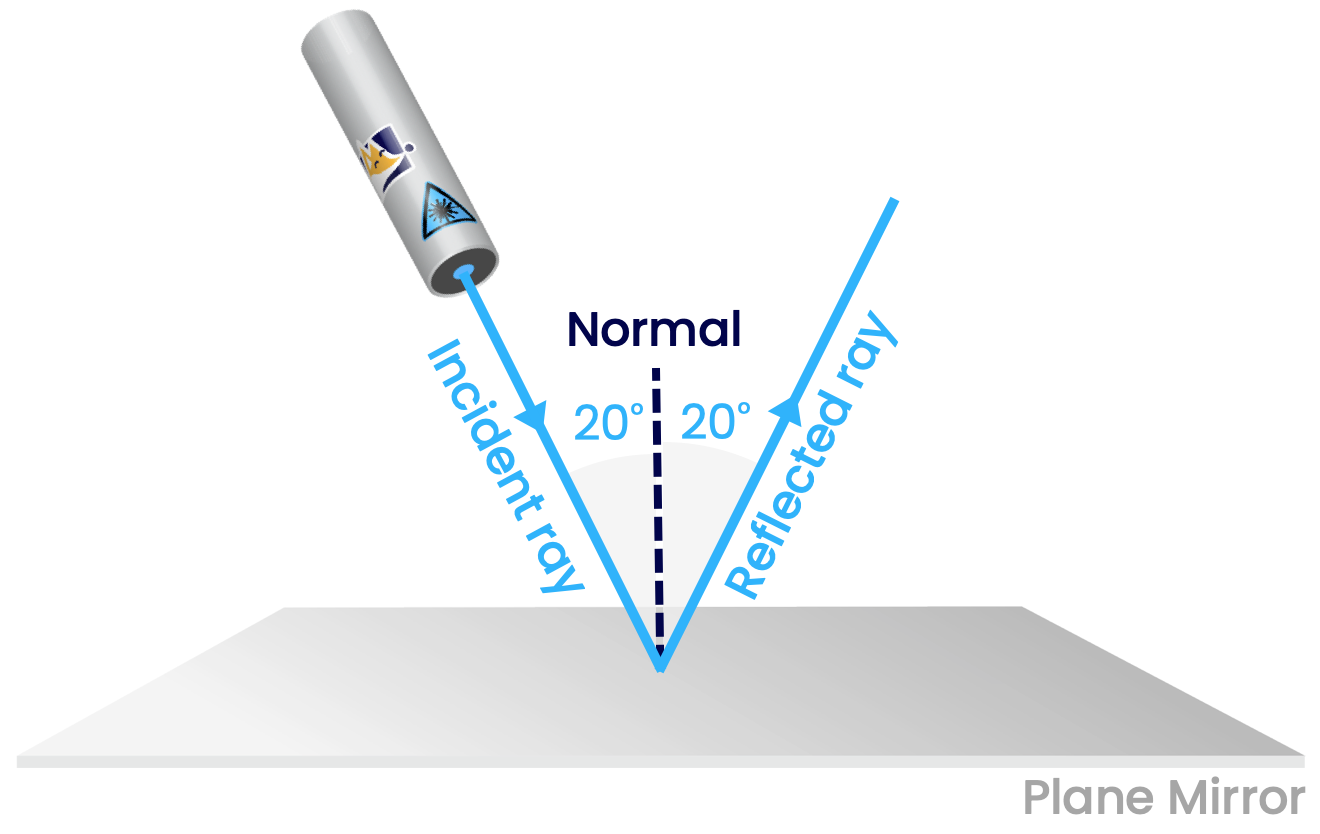Note that the angle is measured between the light ray and the dotted line (NOT between the light ray and the mirror). This dotted line is called the normal, and is always at right-angles to the mirror. The angle between the incident ray and the normal is called the angle of incidence, and the angle reflected ray and the normal is called the angle of reflection.

Okay, this time we'll try a larger angle of incidence: 80o.Finally, let's try one somewhere inbetween; 55o.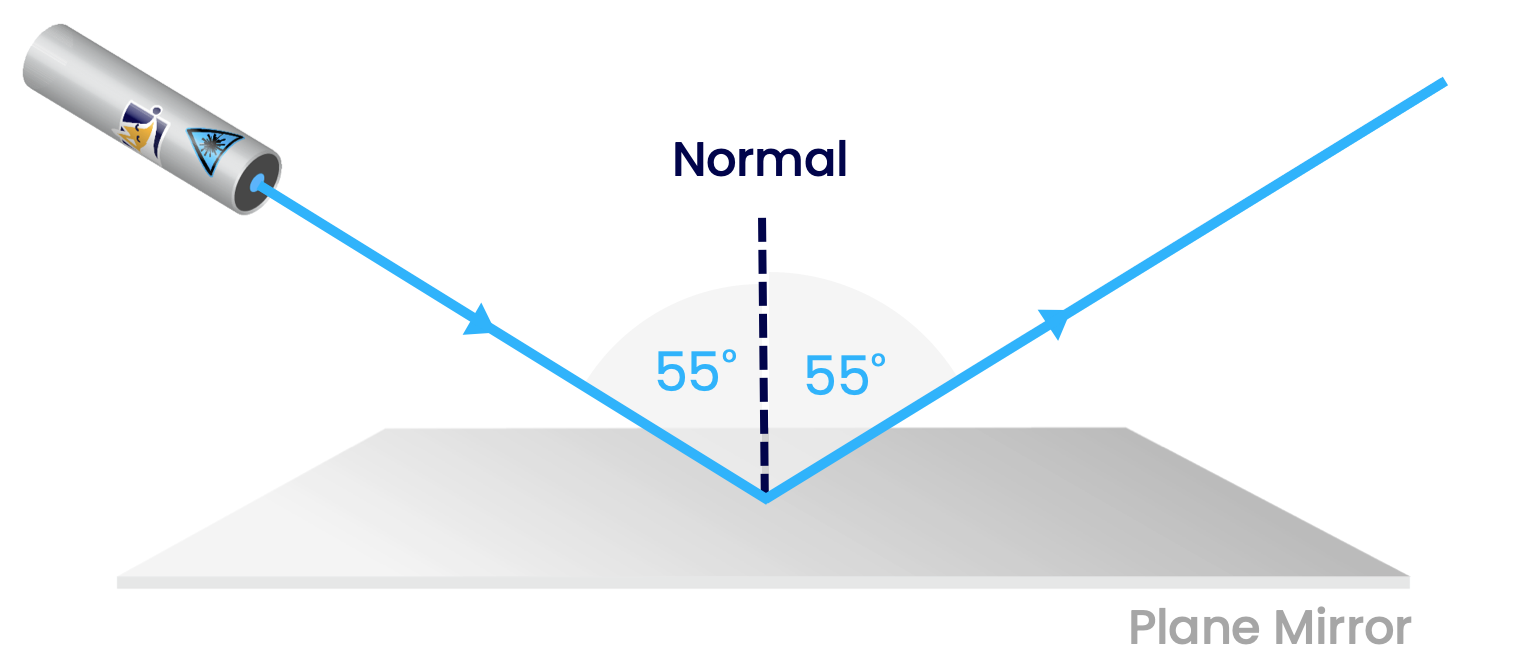Have you noticed anything about the angle of incidence (i) and angle of reflection (r)? They're always the same, regardless of what the angle is!

We can write this as an equation: $i = r$

This is your first equation! We often use equations to describe laws of physics, as it's much quicker than putting it into words. Look how much less space we need to write "i=r", compared to "the angle of incidence is always the same as the angle of reflection" !

However, keep in mind that an equation doesn't actually mean anything unless you know what each symbol means!

The law of reflection doesn't only apply to light! It's also true any time that a ball hits a wall, as seen in the game of pool,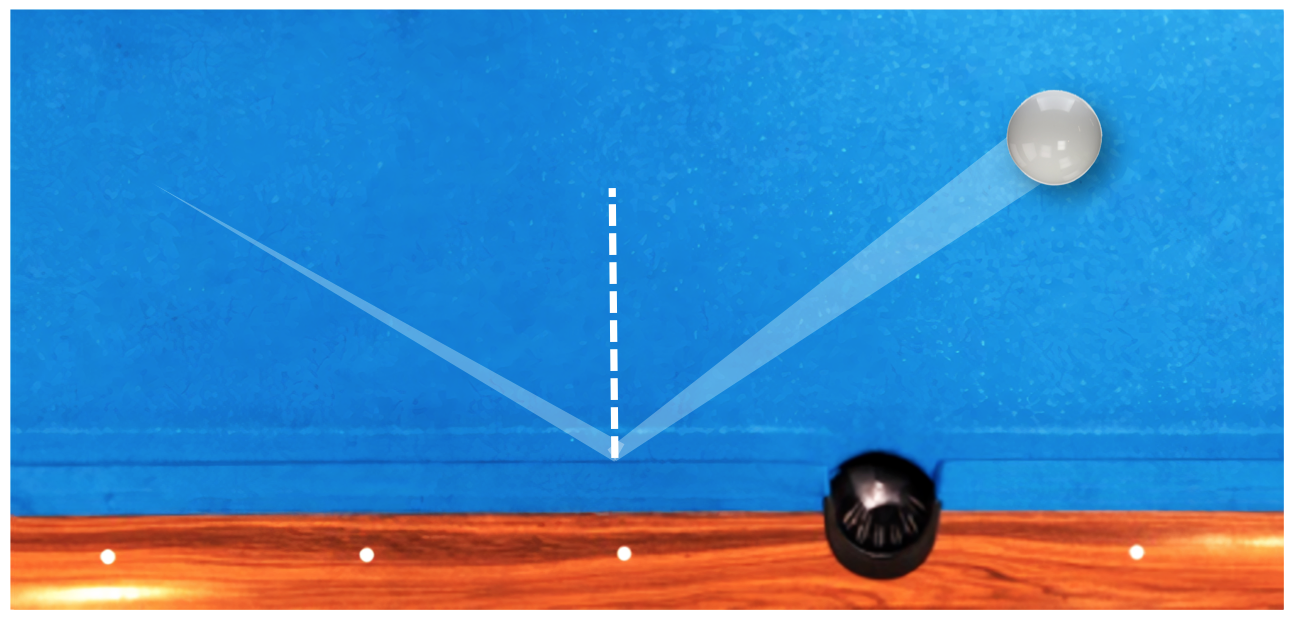and the beautiful game-of-two-halves itself, football.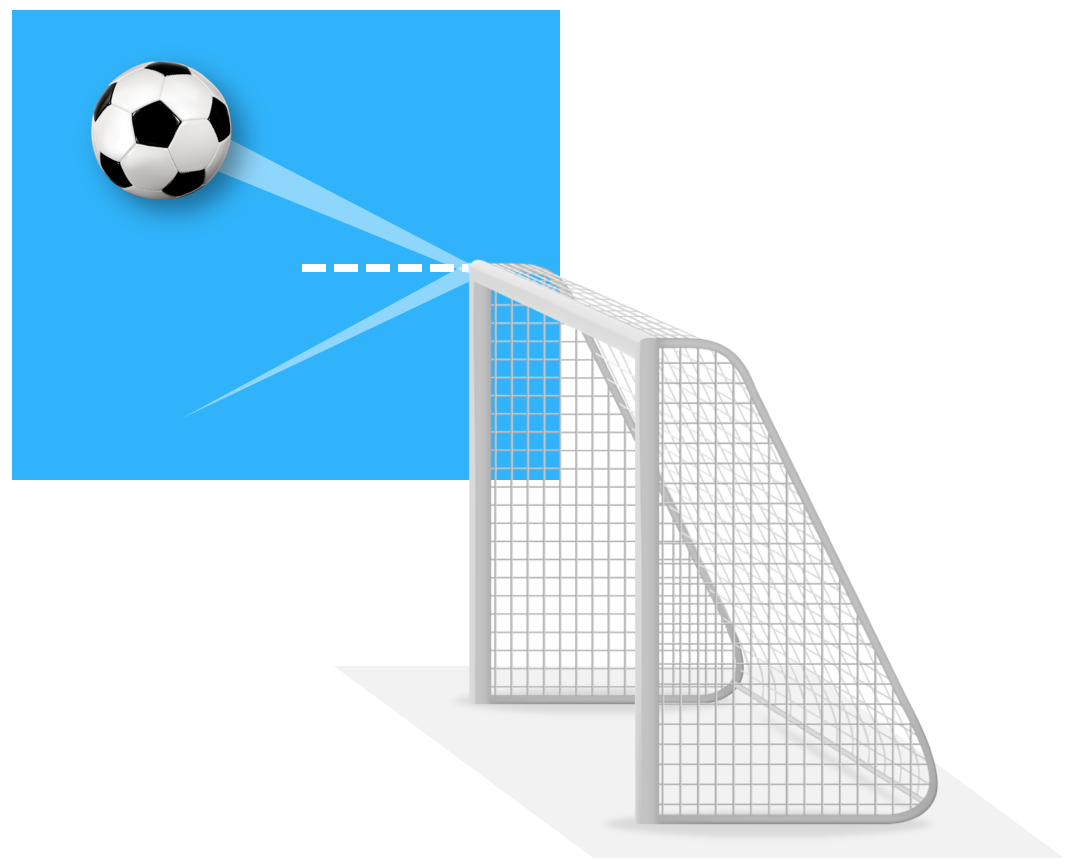Quiz

### Congratulations!

7 of 7 questions completed

+ ⭐️ collected.

• You can change your display name at any time.
• at least 1 letter
• at least 1 number
• at least 8 characters